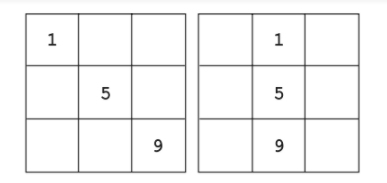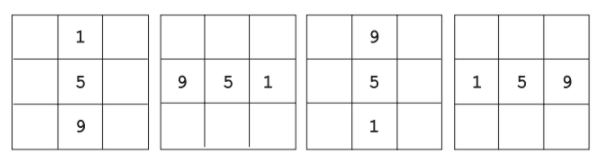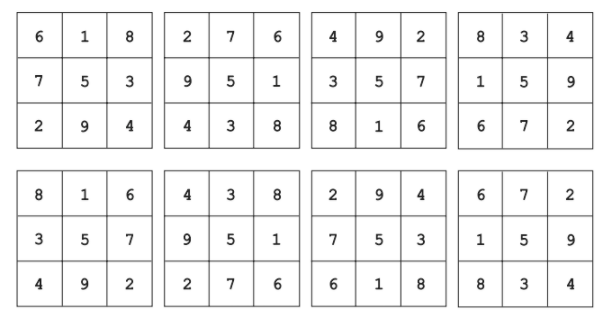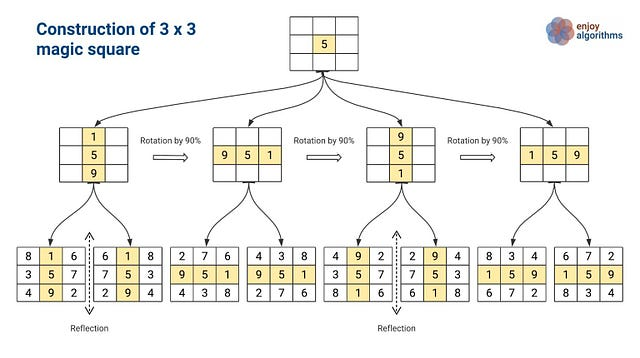# Magic Square Puzzle

### Magic Square Problem Statement

Fill the 3 × 3 tables with nine distinct integers from 1 to 9 so that the sum of the numbers in each row, column, and corner-to-corner diagonal is the same.

### Solution Idea 1: Exhaustive Search

The basic idea would be to explore ways to place numbers 1 to 9 in the table and check the magic square condition. So the critical question is: how many ways are there to fill such a table? Let think!

We can start filling the table with one number at a time: starting with placing the 1 somewhere and ending with placing the 9.

• There are nine unique ways to place 1, followed by eight ways to place 2, and so on until the last number 9 is placed in the only unoccupied cell of the table.
• Hence, there are 9 · 8 · … · 1 = 9! = 362,880 ways to arrange the nine numbers in the cells of the 3 × 3 table.
• Therefore, solving this problem by exhaustive search would imply generating all 362,880 possible arrangements of distinct integers from 1 to 9 in the table and checking, for each of the arrangements, whether all its row, column, and diagonal sums are the same.

If we further increase the value of n, then an exhaustive search algorithm needs to explore many candidate solutions to get the final answer. So this solution is inefficient because the amount of work is impossible to do by hand. Even a computer would take a considerable time to finish the job!

So the critical question is: What would be the efficient way to solve this problem? Can we have some mathematical insight into the problem to solve the problem using the fewer number step? Let's think!

### Efficient Solution Idea

It is not difficult to solve this puzzle by proving two critical constraints of the 3x3 magic square: 1) The value of the common sum will be 15, which is also called magic sum 2) The central cell must contain value 5.

As we know that sum all columns, rows, and diagonals are equal, so the magic sum = sum of all the numbers in its rows divided by the number of rows = sum of all the columns divided by the number of columns = (1 + 2 +··· + 9)/ 3 = 15. So the value of the magic sum is equal to 15.

The second step would be to find the value of the central cell. Suppose the numbers in row 1, row 2, and row 3 are: (a, b, c), (d, e, f), (g, h, i). Here 'e' is the value of the number in the central cell. How can we find this value? Let's think!

As we know, the sum of each row, diagonal, and column will be 15. So we sum the row, column, and diagonal involving the central cell i.e. we add the numbers in the second row, the second column, and the two main diagonals. Here is the equation:

=> (d + e + f ) + (b + e + h) + (a + e + i) + (g + e + c) = 4*15

=> 3e + (a + b + c) + (d + e + f ) + (g + h + i) = 60

=> 3e + sum of numbers from 1 to 9 = 60

=> 3e + 45 = 60

=> e = 5

The central cell must contain value 5, and the sum of the other two values of the row or diagonal or column, including the central cell, must be 10. To achieve the magic sum, we will have four possible pairs of the remaining 8 numbers, which gives the sum equal to 10: (1, 9), (2, 8), (3, 7), and (4, 6). So we need to build the magic square by arranging four pairs of numbers around the central cell. Think! Looking at the table’s symmetries, there are only two different ways to put 1 and 9: in the table’s corners and not in the table’s corners.But placing the numbers 1 and 9 in the table corners cannot form a magic square. Think! Suppose we are placing 1 in the upper left corner and 9 in the bottom right corner. If we put a number less than 5 in the upper right corner, we will not have the magic sum of 15 in the first row. Similarly, if we put a number larger than 5, we will have the same problem with the last column. So we need to ignore tha idea of placing pairs (1, 9) in the table corners.Now we concentrate on the second possibility to put pair (1, 9) around the central row or column in the table. There are four possible ways to put 1 and 9 in the same row or the same column with 5. For each possibility:

• The line containing 1 must be filled with 6 and 8.
• The line containing 1 must be filled with 2 and 4.
• The line not containing 1 or 9 must be filled with 3 and 7.As we can see in the figure, there could be 8 possible 3 x 3 magic squares. For each possible placement of pair (1, 9), we can obtain two different solutions, which are a reflection of each other. Of course, all of them are symmetric and can be obtained from one of the eight by rotation and reflection.

Some critical facts related to the 3x3 magic square:

• The central cell must contain the value 5.
• The value of the magic sum is equal to 15.
• The diagonal arrangement of 1, 5, and 9 is not feasible.
• A line containing 1 must be filled with 6 and 8.
• All 8 solutions of the 3x3 magic square are symmetric and can be obtained by the rotation and reflection of each other### Critical Ideas to Explore!

• The number of different magic squares of order 2x2, 3x3, 4x4, and 5x5 is 0, 1, 880, 275305224 (excluding those obtained by rotation and reflection). The exact count of 6x6 magic squares is not known.
• Since their appearance in ancient India, Japan, and China, the magic squares have fascinated people for thousands of years. One of the unsolved problems is how to design a generic algorithm to generate any magic square of order NxN (N>2).
• One can use several known algorithms to construct magic squares of an arbitrary order n ≥ 3, which are especially efficient for odd n’s. Of course, these algorithms are not based on exhaustive search!

Enjoy learning, enjoy mathematics!